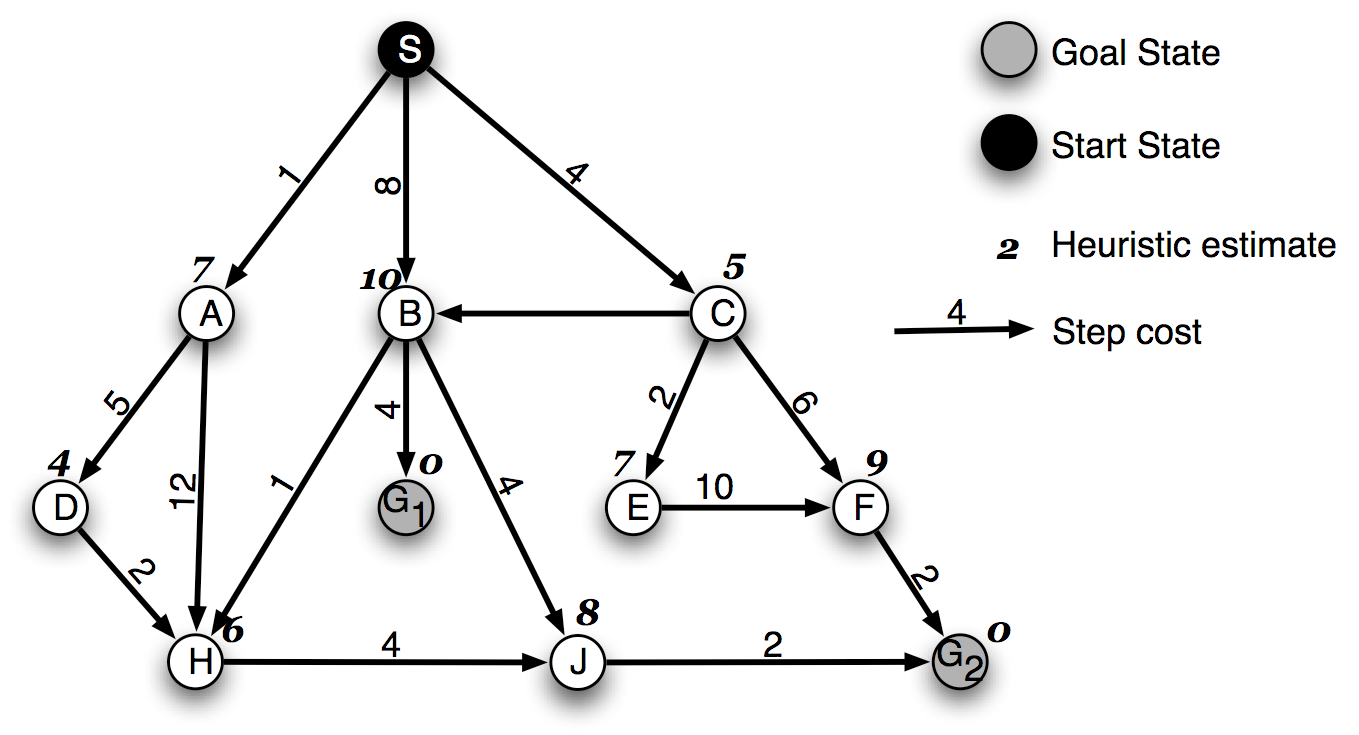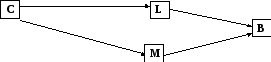# Midterm exam

Download 0,51 Mb.
 Page 2/2 Date conversion 25.04.2017 Size 0,51 Mb.
1   2

3. [20 points] Consider the search space below, where S is the start node and G1 and G2 are goal nodes. Arcs are labeled with the value of a cost function; the number gives the cost of traversing the arc. Above each node is the value of a heuristic function; the number gives the estimate of the distance to the goal. Assume that uninformed search algorithms always choose the left branch first when there is a choice. Assume that the algorithms do not keep track of and recognize repeated states.
For each of the following search strategies,

(a) indicate which goal state is reached first (if any) and

(b) list in order, all the states that are popped off the OPEN list.SOLUTION
Depth-first

(a) G2 (2 pt)

(b) S, A, D, H, J, G2 (2pt)

Iterative Deepening

(a) G1

(b) S, A, B, C, S, A, D, H, H, B, H, G1

Breadth-first

(a) G1

(b) S, A, B, C, D, H, H, G1

Greedy

(a) G2

(b) S, C, A, D, H, E, J, G2

A*

(a) G1

(b) S, A, C, D, E, H, B, G1

SOLUTION
Depth-first

(a) G2

(b) S, A, D, H, J, G2

Iterative Deepening

(a) G1

(b) S, A, B, C, S, A, D, H, H, B, H, G1

Breadth-first

(a) G1

(b) S, A, B, C, D, H, H, G1

Greedy

(a) G2

(b) S, C, A, D, H, E, J, G2

A*

(a) G1

(b) S, A, C, D, E, H, B, G1

SOLUTION
Depth-first

(a) G2

(b) S, A, D, H, J, G2

Iterative Deepening

(a) G1

(b) S, A, B, C, S, A, D, H, H, B, H, G1

Breadth-first

(a) G1

(b) S, A, B, C, D, H, H, G1

Greedy

(a) G2

(b) S, C, A, D, H, E, J, G2

A*

(a) G1

(b) S, A, C, D, E, H, B, G1

Part III. 20 points. 15 minutes.

Short Answer. Answer 4 out of the following 6 problems. Use no more than 2 sentences for each question. Each question is worth 5 points. You may put your answer on the test sheet.

1. Under what conditions does A* produce the optimal solution?

When the heuristic that A* uses is admissible (i.e. an underestimate of the true cost to the goal)

2. Could we apply the Turing test to a computer that plays chess? Why or why not?

If we restricted the questions asked to the chess playing computer to be questions about what is the best move to make next, then we could use the Turing test by comparing the computer’s answers to a human playing the same game. If we allow any questions, then the chess playing computer would most definitely lose the Turing test.
3. How is information gain used to select the best attribute for decision tree learning? Describe metrics (but no need to give formulae) and effect of using information gain on the resulting tree.

Two metrics would be used: entropy and information gain. Entropy measures the homogeneity of a class of examples. If all are the same it returns a value of 0, if they are equally split, it returns a value of 1. In between this, it will return a value between 0 and 1, being closer to 1 if they are more equally split than not. Information gain measures the difference between entropy of the original set and entropy of the subsets obtained after choosing an attribute. The attribute that gives the largest information gain is chosen.

4. Calculate the value of P(C,L,M,B) for the Bayesian net shown below. It is sufficient to show how you would calculate it without actually doing the arithmetic.
 L M P(B=true|L,M) True True ..2 True False .9 False True .3 False False .8
 C P(L=true|C) True .2 False .7

P(C=true) = 0.1 0.20.20.2C P(M=true|C) True .4 False .5

P(C,L,M,B) = .1*.6*.2*.1

5. Describe 3 sources of knowledge that IBM’s Deep Blue uses to select good moves.
Book of opening moves, end game database, and heuristics

6. Describe how hill-climbing is used for machine translation, including the evaluation function that is used to determine the best next state.

We start off with a translation based on individual word translations. This is the initial state. Then the successor function proposes modifications to the initial translation, which may include substituting a word, deleting a word, adding a word, among others. The different possible new translations (note that there are many) are then scored using a language model and the best one is accepted.

1   2

The database is protected by copyright ©sckool.org 2016
send message

Main page